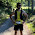## Monday, May 20, 2013

### Validating composite models with knockout validation

When you use knockout validation to extend observables with validation rules, it will add a few functions to these observables - the most important ones being; error and isValid. You can use these functions to verify if any of the validation rules were violated, and to extract an error message.

To extract all of the error messages out of a composite model, you can use a grouping function.
```function BookingModel() {
var self = this;

self.contact = new ContactModel();
self.departure = new DepartureModel();

self.isValid = function() {
return self.contact.isValid() && self.departure.isValid();
};

self.validate = function() {
if (!self.isValid()) {
var errors = ko.validation.group(self);
errors.showAllMessages();

return false;
}

return true;
};
}

function DepartureModel() {
this.street = ko.observable('').extend({ required: true });
this.houseNumber = ko.observable('').extend({ required: true });
this.city = ko.observable('').extend({ required: true });
this.time = ko.observable('').extend({ required: true });

this.isValid = function() {
return
this.street.isValid() &&
this.houseNumber.isValid() &&
this.city.isValid() &&
this.time.isValid();
};
}

function ContactModel() {
this.firstName = ko.observable('').extend({ required: true });
this.lastName = ko.observable('').extend({ required: true });
this.phoneNumber = this.firstName = ko.observable('').extend({ required: true });
this.email = ko.observable('').extend({ required: true });

this.isValid = function() {
return
this.firstName.isValid() &&
this.lastName.isValid() &&
this.phoneNumber.isValid() &&
this.email.isValid();
};
}
```

This is what my first attempt looked like. A little later I discovered that you can get rid of these boilerplate functions on the composite model by applying the validatedObservable function instead.
```ko.applyBindings(ko.validatedObservable(new BookingModel()));

ko.validatedObservable = function (initialValue) {
if (!exports.utils.isObject(initialValue)) {
return ko.observable(initialValue).extend({ validatable: true }); }

var obsv = ko.observable(initialValue);
obsv.errors = exports.group(initialValue);
obsv.isValid = ko.computed(function () {
return obsv.errors().length === 0;
});

return obsv;
};```
The validatedObservable function will add an errors function to the composite model which traverses the object graph and validates each eligible property. The errors function also has a showAllMessages function that will display an error message next to each invalid element. The isValid function only asserts if there are any errors.
```function BookingModel() {
var self = this;

self.contact = new ContactModel();
self.departure = new DepartureModel();

self.validate = function() {
if (!self.isValid()) {
self.errors.showAllMessages();

return false;
}

return true;
};
}

function DepartureModel() {
this.street = ko.observable('').extend({ required: true });
this.houseNumber = ko.observable('').extend({ required: true });
this.city = ko.observable('').extend({ required: true });
this.time = ko.observable('').extend({ required: true });
}

function ContactModel() {
this.firstName = ko.observable('').extend({ required: true });
this.lastName = ko.observable('').extend({ required: true });
this.phoneNumber = this.firstName = ko.observable('').extend({ required: true });
this.email = ko.observable('').extend({ required: true });
}```

Removing that cruft results in less bulky, cheaper models.

If you try this example, you will notice that the model appears to be valid even though the validation rules are clearly violated. It took me a few minutes of browsing the source to figure out why this was happening. When you use the group functions to validate your model, they will by default only look at first level properties. So if you have a composite model, you need to modify the grouping validation configuration, and set the deep property to true.
`ko.validation.init({ grouping : { deep: true, observable: true } });`

1.You can simplify your code even more because you don't need the validatedObservable function.

var bookingModel = new BookingModel();
bookingModel.errors = ko.validation.group(bookingModel, { deep: true });
ko.applyBindings(bookingModel);

1.But then I'm just applying the validatedObservable function by hand?

2.My mistake, I thought you'd written the validatedObservable function yourself. I see what you mean now and I like that approach. Thanks for taking the time to write about it

3.in fact you can use a group for multiple objects :) so yeap, one group can deal with both, but still its not the cleanest solution. And validatedObservable is only a nice way of hiding the group call in fact.

2.very useful..thanks a lot

3.Do you have the HTML for this as well? Maybe post to JSFiddle?

4.How many levels it recognize?

5.Hello Jef,